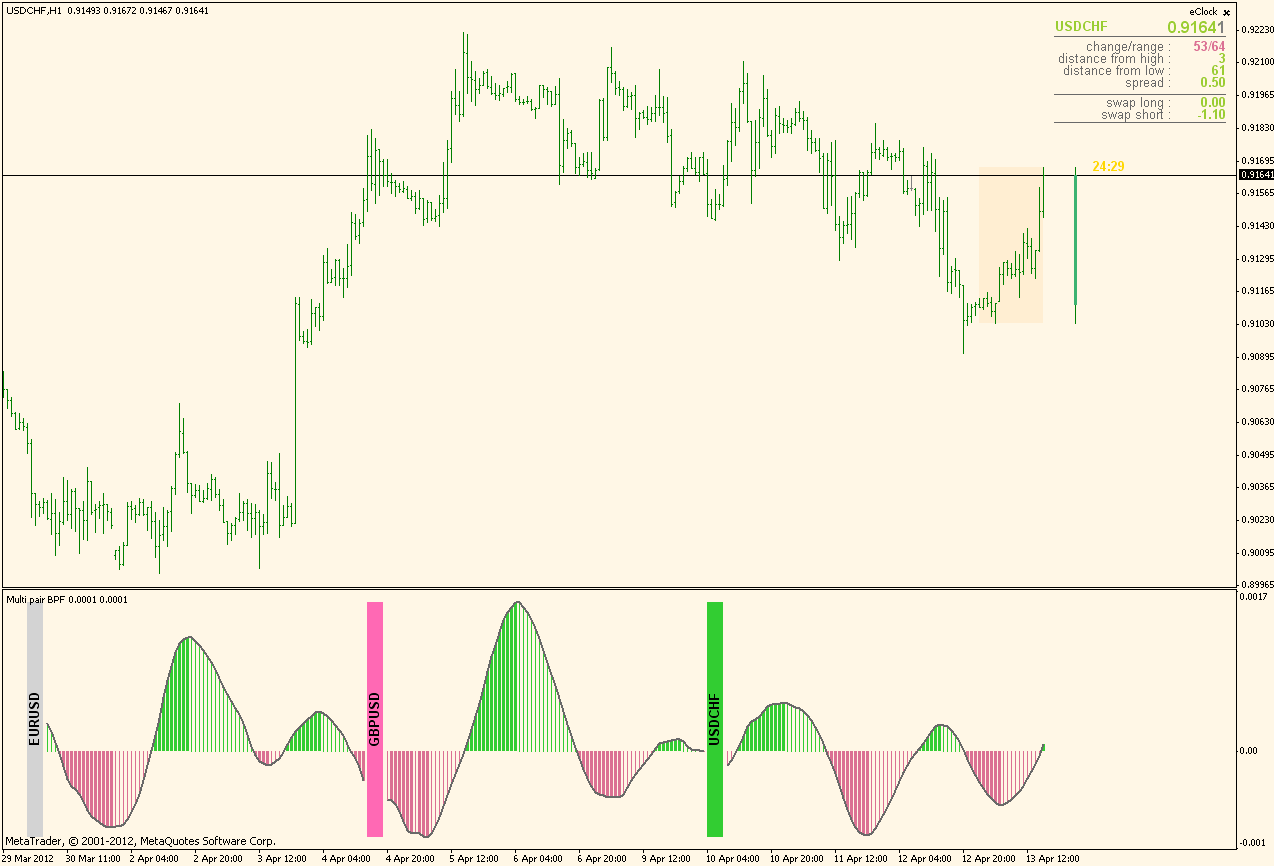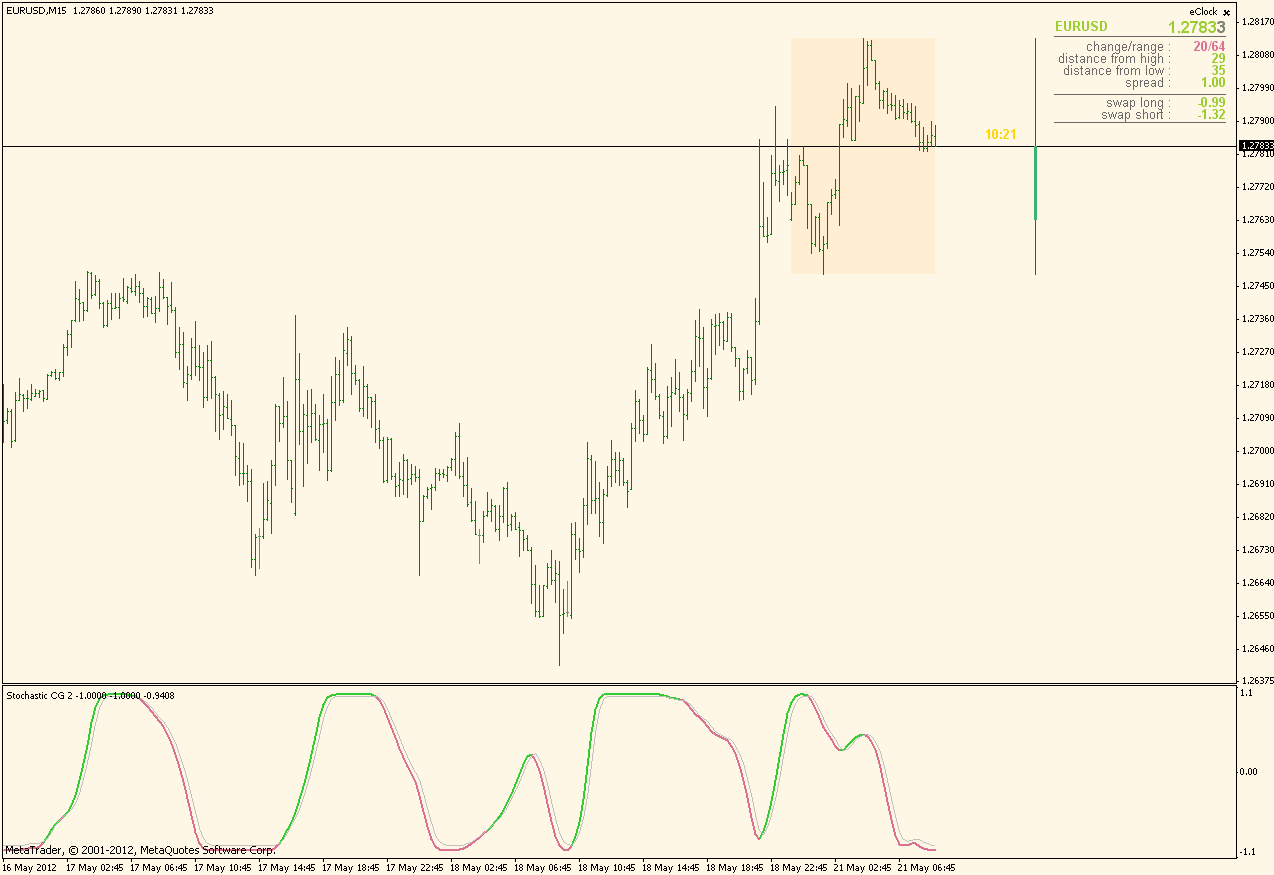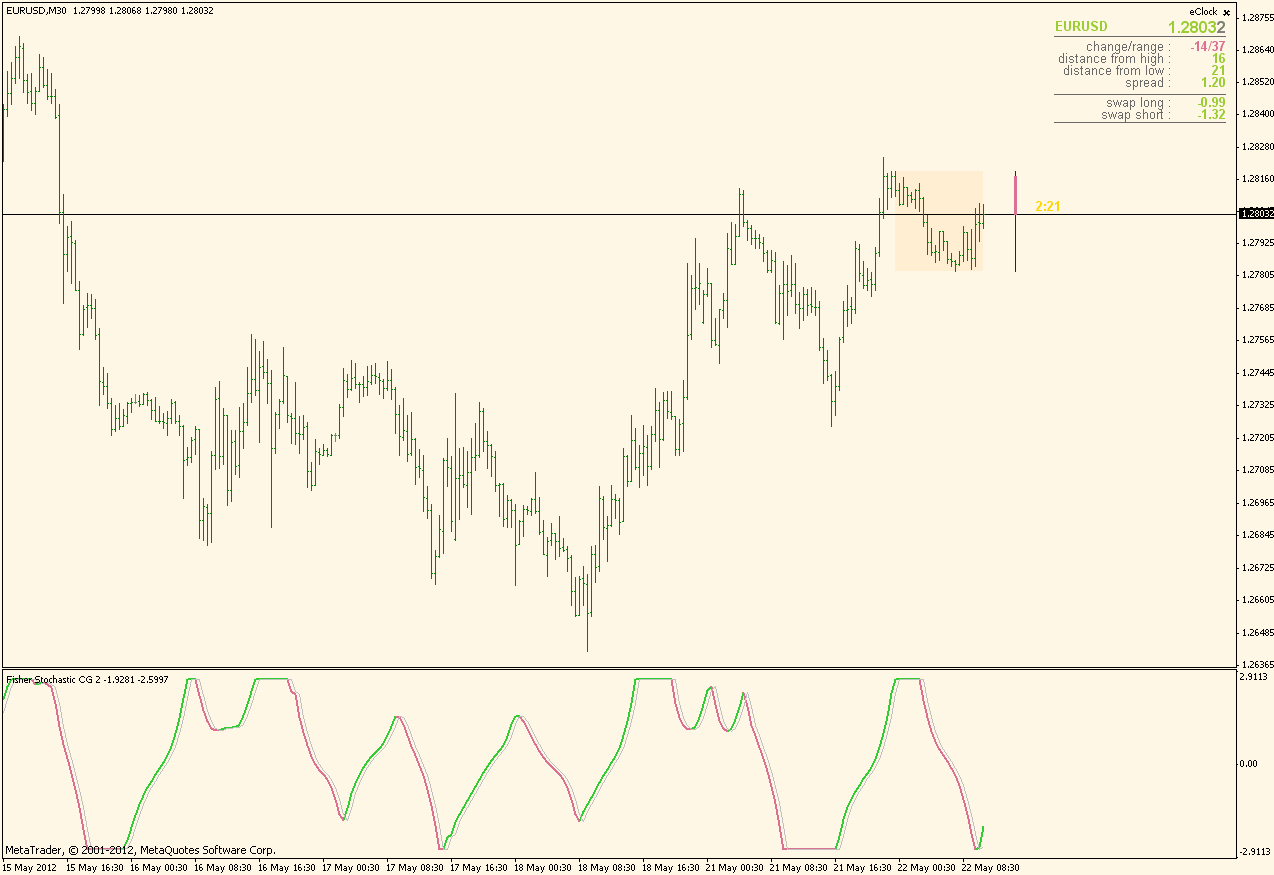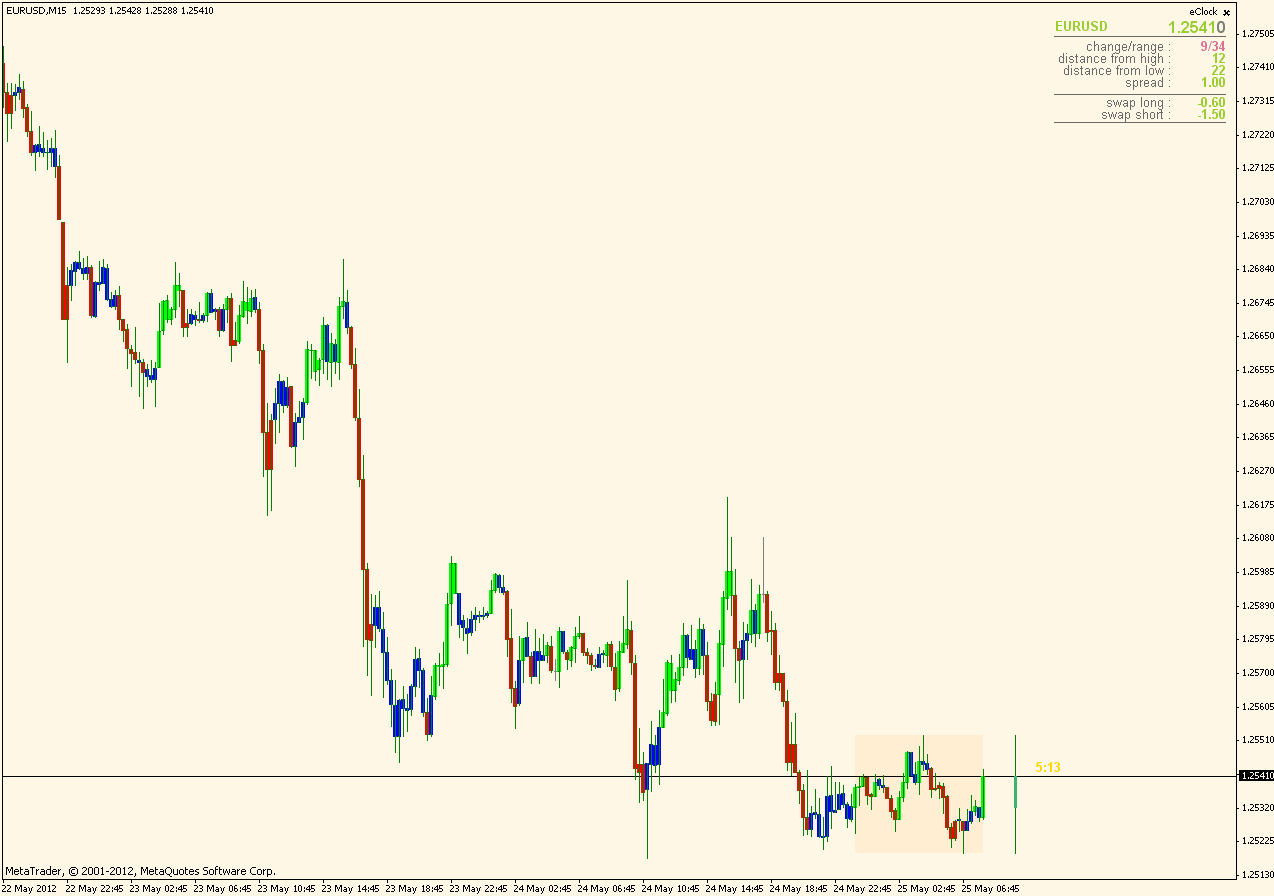• Get access

# All John Ehlers Indicators... - page 43163199

Multi pair band pass filter ...

Multi pair band pass filter posted at this post : https://www.mql5.com/en/forum/178416/page12Files:165

Blau ECO devergence indicator

May we have a Blau ECO divergence indicator?163199

Blau ergodic DI ...

Posted it here : https://www.mql5.com/en/forum/173131/page3

skn:
May we have a Blau ECO divergence indicator?163199

Stochastic center of gravity ...

There is one similar to this indicator already at this thread, but this one is simplified, made exactly as it is described by John Ehlers and added some functionality (color changes on trend changes) so it can be considered as a sort of an upgrade of the Stochastic CG (stochastic center of gravity)Files:
sto_cg.gif 24 kb163199

Fisher stochastic CG ...

Almost the same as previous except that the result is "fisherized" That way it can be a bit more responsive and easier to spot trend changes. Colors are changing on slope change in this oneFiles:3

Elder Impulse Candles

Hi Guys thanks fo the indicators. Surely womderful. I tried the Impulse candle . But it doen't work. Any one any ideas?

Km

Gramski:
Hi all...

I'm dedicating this thread to all indicators by John Ehler's in the hope that we can get/make as many of them for MT4 as possible.

Please post any MT4 John Ehlers indicators

that you have here as outlined in his books "Rocket Science for Traders", "Cybernetic Analysis for Stocks and Futures", "Mesa and Trading Market Cycles".

Please post any coding for indicators that programmers could convert to Mql4.

Thanks to Igorad and others for already programming many of these indicators.

To kick things off see my attachments...

Gramski.163199

...

If you are refering to this indicator https://c.mql5.com/forextsd/forum/49/elder_impulse_candle_color.mq4 (from this thread, at this post : https://www.mql5.com/en/forum/174980/page10), this is how it looks on my terminal :Hi Guys thanks fo the indicators. Surely womderful. I tried the Impulse candle . But it doen't work. Any one any ideas? Km
Files:279

Hi,

I'm looking for the sinewave indicator from John Ehlers using the weighted moving average. This is the one from his book "Rocket Science for traders", and not the one from "Cybernetic analysis...". Anybody knows where I could find it?13

Hi, this is code for indicator called "Ehlers Dominant Cycle Period". It shuld return the length of dominant cyle in the markets. Is this indicator available asi mq4? Maybe it has been posted here under different name.

Indicator: EhlersDominantCycle

inputs:

Price( MedianPrice ) ;

variables:

alpha1( 0 ),

alpha1Plus1( 0 ),

Log10( 0 ),

HP( 0 ),

SmoothHP( 0 ),

EhlersDelta( 0.1 ),

EhlersBeta( 0 ),

Cos720Delta( 0 ),

EhlersGamma( 0 ),

alpha( 0 ),

OneMinusAlpha( 0 ),

OnePlusAlpha( 0 ),

N( 0 ),

TwoPi( 6.2831854 ),

MaxAmpl( 0 ),

Num( 0 ),

Denom( 0 ),

DC( 0 ),

DomCyc( 0 ),

SineLine( 0 ),

CosineLine( 0 ) ;

arrays:

EhlersI( 0 ),

OldI( 0 ),

OlderI( 0 ),

Q( 0 ),

OldQ( 0 ),

OlderQ( 0 ),

Real( 0 ),

OldReal( 0 ),

OlderReal( 0 ),

Imag( 0 ),

OldImag( 0 ),

OlderImag( 0 ),

Ampl( 0 ),

OldAmpl( 0 ),

DB( 0 ) ;

if CurrentBar = 1 then

begin

{ 360 / 40 = 9 }

alpha1 = ( 1 - Sine( 9 ) ) / Cosine( 9 ) ;

alpha1Plus1 = alpha1 + 1 ;

Log10 = Log( 10 ) ;

end ;

HP = 0.5 * alpha1Plus1 * ( Price - Price ) +

alpha1 * HP ;

SmoothHP = ( HP + 2 * HP + 3 * HP + 3 * HP +

2 * HP + HP ) / 12 ;

if CurrentBar = 1 then

SmoothHP = 0

else if CurrentBar < 7 then

SmoothHP = Price - Price ;

EhlersDelta = -0.015 * CurrentBar + 0.5 ;

EhlersDelta = MaxList( 0.15, EhlersDelta ) ;

if CurrentBar > 6 then

begin

for N = 8 to 50

begin

EhlersBeta = Cosine( 360 / N ) ;

Cos720Delta = Cosine( 720 * EhlersDelta / N ) ;

if Cos720Delta 0 then

EhlersGamma = 1 / Cos720Delta ;

alpha = EhlersGamma -

SquareRoot( Square( EhlersGamma ) - 1 ) ;

OneMinusAlpha = 1 - alpha ;

OnePlusAlpha = 1 + alpha ;

Q[N] = ( N / TwoPi ) * ( SmoothHP -

SmoothHP ) ;

EhlersI[N] = SmoothHP ;

Real[N] = 0.5 * OneMinusAlpha * ( EhlersI[N] -

OlderI[N] ) + EhlersBeta * OnePlusAlpha *

OldReal[N] - alpha * OlderReal[N] ;

Imag[N] = 0.5 * OneMinusAlpha * ( Q[N] -

OlderQ[N] ) + EhlersBeta * OnePlusAlpha *

OldImag[N] - alpha * OlderImag[N] ;

Ampl[N] = ( Square( Real[N] ) +

Square( Imag[N] ) ) ;

end ;

end ;

for N = 8 to 50

begin

OlderI[N] = OldI[N] ;

OldI[N] = EhlersI[N] ;

OlderQ[N] = OldQ[N] ;

OldQ[N] = Q[N] ;

OlderReal[N] = OldReal[N] ;

OldReal[N] = Real[N] ;

OlderImag[N] = OldImag[N] ;

OldImag[N] = Imag[N] ;

OldAmpl[N] = Ampl[N] ;

end ;

MaxAmpl = Ampl ;

for N = 8 to 50

begin

if Ampl[N] > MaxAmpl then

MaxAmpl = Ampl[N] ;

end ;

for N = 8 to 50

begin

if MaxAmpl 0 and ( Ampl[N] / MaxAmpl ) > 0 then

DB[N] = -10 * Log( 0.01 / ( 1 - .99 * Ampl[N] /

MaxAmpl ) ) / Log10 ;

if DB[N] > 20 then

DB[N] = 20 ;

end ;

Num = 0 ;

Denom = 0 ;

for N = 10 to 50

begin

if DB[N] <= 3 then

begin

Num = Num + N * ( 20 - DB[N] ) ;

Denom = Denom + ( 20 - DB[N] ) ;

end ;

if Denom 0 then

DC = Num / Denom ;

end ;

DomCyc = Median( DC, 10 ) ;

if DomCyc < 8 then

DomCyc = 20 ;

EhlersBeta = Cosine( 360 / DomCyc ) ;

Cos720DeltaDom = Cosine( 720 * EhlersDelta / DomCyc ) ;

EhlersGamma = 1 / Cos720DeltaDom ;

alpha = EhlersGamma - SquareRoot( Square( EhlersGamma )

- 1 ) ;

SineLine = 0.5 * ( 1 - alpha ) * ( SmoothHP -

SmoothHP ) + EhlersBeta * ( 1 + alpha ) *

SineLine - alpha * SineLine ;

CosineLine = ( DomCyc / TwoPi ) * ( SineLine -

SineLine ) ;

if CurrentBar > 10 then

begin

Plot1( SineLine, "Sine", Red, default, 2 ) ;

Plot2( CosineLine, "Cosine", Cyan, default, 2 ) ;

end ;80

Fama quits painting

Calling a coder, Mladen maybe *-)

This Fama Indy stops painting on current chart, and someone has to change TF before it shows the full line again.

Maybe someone can find and fix the big in the code?

TIA

Files:
fama.mq4 4 kb